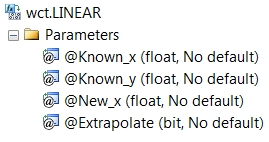# SQL Server linear interpolation with extrapolation

LINEAR

Updated: 20 November 2013

Note: This documentation is for the SQL2008 (and later) version of this XLeratorDB function, it is not compatible with SQL Server 2005.
Click here for the SQL2005 version of the LINEAR function

Use the aggregate function LINEAR to calculate the straight-line interpolated value of y given x in the array (x, y). LINEAR is not sensitive to the order of the array (x, y). LINEAR supports extrapolation when the new x-value is outside the bounds of the supplied x-values or permits you to return the y-value associated with maximum x-value or the y-value associated with the minimum x-value when the new x-value is greater than the maximum x-value or less than the minimum x-value.
SyntaxArguments
@Known_x
the x-values to be used in the interpolation calculation. @Known_x must be of the type float or of a type that implicitly converts to float.
@Known_y
the y-values to be used in the interpolation calculation. @Known_y must be of the type float or of a type that implicitly converts to float.
@New_x
the new x-value for which you want LINEAR to calculate the y-value. @New_x must be of the type float or of a type that implicitly converts to float.
@Extrapolate
a bit value that identifies whether to return the boundary value or the extrapolated value when @New_x is outside the boundary. @Extrapolate must be of a type bit or of a type that implicitly converts to bit.
Return Types
float
Remarks
·         For cubic spline interpolation use the SPLINE function.
·         For polynomial interpolation, use the POLYINTERP function.
·         For monotonic spline interpolation use the MONOSPLINE function.
·         For bilinear interpolation use the INTERP2 function.
·         If @New_x is less than the smallest x-value and @Extrapolate is TRUE, then an extrapolated value is returned, otherwise the y-value paired with the smallest x-value is returned.
·         If @New_x is greater than the largest x-value and @Extrapolate is TRUE, then an extrapolated value is returned, otherwise the y-value paired with the largest x-value is returned.
·         If @Extrapolate is NULL then @Extrapolate is equal to FALSE.
·         If an x-y pair contains a NULL, then the pair is not used in the interpolation calculation.
·         If @New_x = @Known_x then @Known_y is returned.
·         @New_x must remain invariant for the GROUP.
·         @Extrapolate must remain invariant for the GROUP.
·         The number of rows passed into the function is less than 2 then a NULL is returned.
·         If @New_x is NULL then a NULL is returned.
Examples
Given the following x- and y-values, we want to calculate the y-value for x = 0.75
SELECT wct.LINEAR(x,y,0.75,NULL) as LINEAR
FROM (VALUES
(0.5,0.479426),
(1,0.841471),
(1.5,0.997495),
(2,0.909297),
(2.5,0.598472),
(3,0.14112),
(3.5,-0.350783),
(4,-0.756802)
) n(x,y)

This produces the following result.
LINEAR
----------------------
0.6604485

In this example we calculate the interpolated values with x-values below the minimum and above the maximum with @Extrapolate set to FALSE.
WITH mycte AS (
SELECT *
FROM (VALUES
(0.5,0.479426),
(1,0.841471),
(1.5,0.997495),
(2,0.909297),
(2.5,0.598472),
(3,0.14112),
(3.5,-0.350783),
(4,-0.756802)
) n(x,y)
)
SELECT m.x
,wct.LINEAR(mycte.x, mycte.y,m.x,'False') as LINEAR
FROM (VALUES
(-0.5)
,(0)
,(4.5)
,(5.0)
)m(x), mycte
GROUP BY m.x

This produces the following result.
x                 LINEAR
---------------------- ----------------------
-0.5               0.479426
0               0.479426
4.5              -0.756802
5              -0.756802

Using the same data, but setting @Extrapolate to TRUE.
WITH mycte AS (
SELECT *
FROM (VALUES
(0.5,0.479426),
(1,0.841471),
(1.5,0.997495),
(2,0.909297),
(2.5,0.598472),
(3,0.14112),
(3.5,-0.350783),
(4,-0.756802)
) n(x,y)
)
SELECT m.x
,wct.LINEAR(mycte.x, mycte.y,m.x,'True') as LINEAR
FROM (VALUES
(-0.5)
,(0)
,(4.5)
,(5.0)
)m(x), mycte
GROUP BY m.x

This produces the following result.
x                 LINEAR
---------------------- ----------------------
-0.5              -0.244664
0               0.117381
4.5              -1.162821
5               -1.56884

In this example, we will interpolate using dates.

SELECT wct.LINEAR(
CAST(CAST(x as datetime) as float)
,y
,CAST(CAST('2012-Oct-31' as datetime) as float)
,NULL) as LINEAR
FROM (VALUES
('2012-Apr-30',0.0028),
('2013-Apr-30',0.0056),
('2014-Apr-30',0.0085),
('2016-Apr-30',0.0164),
('2018-Apr-30',0.0235),
('2021-Apr-30',0.0299),
('2031-Apr-30',0.0382),
('2041-Apr-30',0.0406)
) m(x, y)

This produces the following result.
LINEAR
----------------------
0.00421150684931507

### SupportCopyright 2008-2019 Westclintech LLC         Privacy Policy        Terms of Service